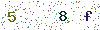﻿ The relationship of LED lighting and power factor - LISUNLISUN

# The relationship of LED lighting and power factor

When the alternating current through by the load, the AC voltage on the load and AC current generation phase difference, people put forward power factor from this concept. As ac load there are three types: resistor, inductor, capacitor:

1. When the alternating current through by pure resistive load, the ac voltage on the resistance with the alternating current are in the same phase position which means the phase angle between them ф= 0°, at the same time, consume active power on resistive load，and the power grid provide energy.

2. When alternating current through by pure inductance load，the phase difference of ac voltage on load is advance of alternating current phase 90 °，the angle between them is ф = 90 °, producing reactive power in the inductive load, electrical energy in the inductance will turn into magnetic field can short storage and then feedback to the power grid become electrical energy, like this cycle, as a result, the power grid is not provide energy, so called “reactive power”, but “reactive current” of “reactive power” still exist.

3. When alternating current by pure capacitance load, also like this, but the phase of ac voltage lag of alternating current phase 90 °, the angle between them is ф = – 90 °.

When alternating current through the perceptual load, ac voltage phase advance of alternating current phase (0 ° ф < 90 °); Alternating current through by capacitive load, the ac voltage phase lag of alternating current phase (- 90 ° ф < 0 °); Electrotechnics define this angle as the power factor angle, the angle cosine value of the power factor angle called power factor. For resistive load, the phase difference the voltage and current is 0 °, therefore, the power factor of the circuit is 1, the largest (Cos 0 ° = 1), and the voltage is advance of electric current; In the pure capacitance, the phase difference of voltage and current is – 90 °, the current is advance of voltage. In the latter two circuits, the power factor is zero (Cos 90 ° = 0). For general load circuit, the power factor is between 0 and 1. By mathematical formula impedance Z = R + j (XL – XC), if XL = XC, Z = R, namely the impedance Z turn into a pure resistance, power factor is equal to 1.